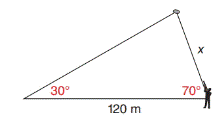Chapter 11.4, Problem 34E### Elementary Geometry for College St...

6th Edition
Daniel C. Alexander + 1 other
ISBN: 9781285195698

#### Solutions

Chapter
Section### Elementary Geometry for College St...

6th Edition
Daniel C. Alexander + 1 other
ISBN: 9781285195698
Textbook Problem
1 views

# In Exercises 29 to 34, use the Law of Sines or the Law of Cosines to solve each problem. Angle measures should be found to the nearest degree and areas and distances to the nearest tenth of a unit.Clay pigeons are released at an angle of 30 ∘ with the horizontal. Massimo hits one of the clay pigeons when shooting through an angle of elevation of 70 ∘ . If the point of release is 120 m from Massimo, how far ( x ) is he from the target when it is hit?To determine

To find:

The distance from the Massimo to the target.

Explanation

Formula:

The Law of Sines,

sinαa=sinβb=sinγc

Where α, β, and γ is the acute angle of the triangle, and a, b, and c is the sides of the triangle.

Calculation:

Given,

Clay pigeons are released at an angle of 30 with the horizontal. Massimo hits one of the clay pigeons when shooting through an angle of elevation of 70.

Let C be the Clay pigeons position and B be the Massimo’s position,

Clay pigeons C are released at an angle of 30 with the horizontal AB

Then A=30 and B=70

Here, AB¯=c=120 m, BC¯=a=x,

A=30andB =70

(i.e.) b=120 m., a=x, α=30 and β=70

Find x:

For any triangle,

α+β+γ=180

30+70+γ=180

100+γ=180<

### Still sussing out bartleby?

Check out a sample textbook solution.

See a sample solution

#### The Solution to Your Study Problems

Bartleby provides explanations to thousands of textbook problems written by our experts, many with advanced degrees!

Get Started

#### Given that the point P(2, 3) lies on the line 2x + ky + 10 = 0, find k.

Applied Calculus for the Managerial, Life, and Social Sciences: A Brief Approach

#### The graph of is: a) b) c) d)

Study Guide for Stewart's Multivariable Calculus, 8th

#### In Exercises 116, determine whether the argument is valid. pq~pq

Finite Mathematics for the Managerial, Life, and Social Sciences